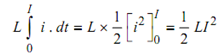## Energy Stored in an Inductor Assignment Help

Assignment Help: >> Inductance - Energy Stored in an Inductor

Energy Stored in an Inductor:

Assume current through the coil of inductance L henry increases by di amperes in dt seconds then

Induced emf = L( di / dt) VoltsEnergy absorbed by magnetic field during time dt second is

iL . (di / dt ). dt = Li . di joules

Therefore, total energy absorbed while current increases from O to I amperes is

W =( ½) LI 2